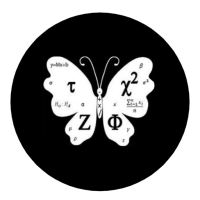## Welcome to Moore Statistics Consulting LLCThe mean symbolizes a typical value in your data. For instance, a mean of 10 would represent that on average ’10’ is the value more or less for all of your data points (unless you have outliers). In terms of discrete random variables, we can express a ‘mean’ using the expected value. If you have a probability of obtaining a particular whole number, you can multiply that probability by your sample size- and obtain an expected value. For example, if I have an event A that occurs half the time and my sample size equals 50 then my expected value becomes 25 (probability times X).

Now usually a expected value problem won’t stop at just one event. You may be given 4 or 5 different probabilities in a ‘grid’ or distribution table. The sum of all the probabilities should equal 1.   Discrete random variables deal with frequencies when you are determining predictions of a typical value.

-moore to follow-Amy